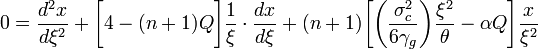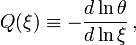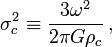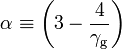$~0 = \frac{d^2x}{d\xi^2} + \biggl[ 4 - (n+1) Q \biggr] \frac{1}{\xi} \cdot \frac{dx}{d\xi} + (n+1) \biggl[ \biggl( \frac{\sigma_c^2}{6\gamma_g } \biggr) \frac{\xi^2}{\theta} - \alpha Q\biggr] \frac{x}{\xi^2}$ where:$~Q(\xi) \equiv - \frac{d\ln\theta}{d\ln\xi} \, ,$$~\sigma_c^2 \equiv \frac{3\omega^2}{2\pi G\rho_c} \, ,$     and,$~\alpha \equiv \biggl(3 - \frac{4}{\gamma_\mathrm{g}}\biggr)$